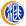# 如何辨别绿钻802

802绿钻是1980年版2元纸币的特殊荧光效果，在荧光灯的照射下，会发出绿色荧光的“田”字图案，看上去像一粒粒钻石，“绿钻”由此得名。500)this.width=500 align=center hspace=10 vspace=10 rel=nofollow/>

500)this.width=500 align=center hspace=10 vspace=10 rel=nofollow/500)this.width=500 align=center hspace=10 vspace=10 rel=nofollow/>

500)this.width=500 align=center hspace=10 vspace=10 rel=nofollow/

802绿钻是在绿幽灵之后被发现的，一开始也被币商命名为“绿幽灵”。后来大众对这款荧光的认可度逐渐升高，直到2018年，PMG才认证了802绿钻为新版别。甚至还传出了“70万成交一捆802绿钻”的新闻引发藏友的关注，据说是首发冠IO。500)this.width=500 align=center hspace=10 vspace=10 rel=nofollow/>

500)this.width=500 align=center hspace=10 vspace=10 rel=nofollow/其实，这些发出荧光的“绿钻”是无意识下的产物，并非刻意设计的，而是当时的一种特殊荧光效果，是一种防伪标识。500)this.width=500 align=center hspace=10 vspace=10 rel=nofollow/>

500)this.width=500 align=center hspace=10 vspace=10 rel=nofollow/500)this.width=500 align=center hspace=10 vspace=10 rel=nofollow/>

500)this.width=500 align=center hspace=10 vspace=10 rel=nofollow/500)this.width=500 align=center hspace=10 vspace=10 rel=nofollow/>

500)this.width=500 align=center hspace=10 vspace=10 rel=nofollow/500)this.width=500 align=center hspace=10 vspace=10 rel=nofollow/>

500)this.width=500 align=center hspace=10 vspace=10 rel=nofollow/如今的五版币中，已经不存在2元面值的纸币，已经退市的四版币2元可以说是2元券的关门币种。加上802在流通时期，消耗非常严重，目前保存下来的极少。而绿钻只占普通802的五分之一或者更少，因此802绿钻的存世量要比想象中的要少很多。500)this.width=500 align=center hspace=10 vspace=10 rel=nofollow/>

500)this.width=500 align=center hspace=10 vspace=10 rel=nofollow/存世量少、双面雕刻、关门绝版币、精美的荧光效果，这些特点集中在一起，使得802绿钻在整个钱币收藏圈也是独一无二的品种，未来潜力不可估量！